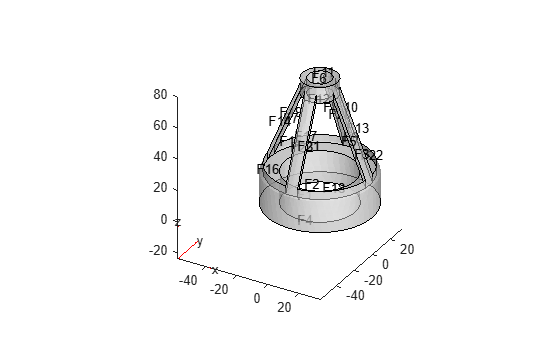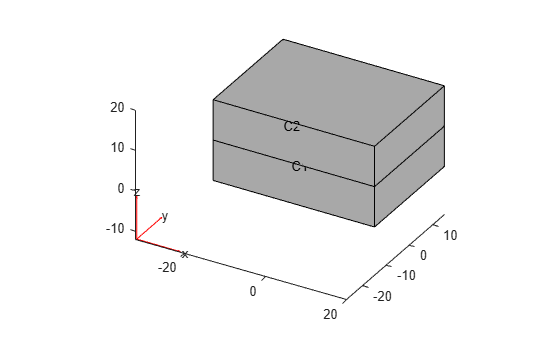# geometryFromMesh

Create 2-D or 3-D geometry from mesh

## Syntax

``geometryFromMesh(model,nodes,elements)``
``geometryFromMesh(model,nodes,elements,ElementIDToRegionID)``
``````[G,mesh] = geometryFromMesh(model,nodes,elements)``````

## Description

example

````geometryFromMesh(model,nodes,elements)` creates geometry within `model`. For planar and volume triangulated meshes, this function also incorporates `nodes` in the `model.Mesh.Nodes` property and `elements` in the `model.Mesh.Elements` property. To replace the imported mesh with a mesh having a different target element size, use `generateMesh`.If `elements` represents a surface triangular mesh that bounds a closed volume, then `geometryFromMesh` creates the geometry, but does not incorporate the mesh into the corresponding properties of the model. To generate a mesh in this case, use `generateMesh`.```

example

````geometryFromMesh(model,nodes,elements,ElementIDToRegionID)` creates a multidomain geometry. Here, `ElementIDToRegionID` specifies the subdomain IDs for each element of the mesh.```
``````[G,mesh] = geometryFromMesh(model,nodes,elements)``` returns a handle `G` to the geometry in `model.Geometry`, and a handle `mesh` to the mesh in `model.Mesh`.```

## Examples

collapse all

Import a tetrahedral mesh into a PDE model.

Load a tetrahedral mesh into your workspace. The `tetmesh` file ships with your software. Put the data in the correct shape for `geometryFromMesh`.

```load tetmesh nodes = X'; elements = tet';```

Create a PDE model and import the mesh into the model.

```model = createpde(); geometryFromMesh(model,nodes,elements);```

View the geometry and face numbers.

`pdegplot(model,'FaceLabels','on','FaceAlpha',0.5)`Create a geometric block from the convex hull of a mesh grid of points.

Create a 3-D mesh grid.

`[x,y,z] = meshgrid(-2:4:2);`

Create the convex hull.

```x = x(:); y = y(:); z = z(:); K = convhull(x,y,z);```

Put the data in the correct shape for `geometryFromMesh`.

```nodes = [x';y';z']; elements = K';```

Create a PDE model and import the mesh.

```model = createpde(); geometryFromMesh(model,nodes,elements);```

View the geometry and face numbers.

`pdegplot(model,'FaceLabels','on','FaceAlpha',0.5)`Create a 3-D geometry using the MATLAB® `alphaShape` function. First, create an `alphaShape` object of a block with a cylindrical hole. Then import the geometry into a PDE model from the `alphaShape` boundary.

Create a 2-D mesh grid.

```[xg,yg] = meshgrid(-3:0.25:3); xg = xg(:); yg = yg(:);```

Create a unit disk. Remove all the mesh grid points that fall inside the unit disk, and include the unit disk points.

```t = (pi/24:pi/24:2*pi)'; x = cos(t); y = sin(t); circShp = alphaShape(x,y,2); in = inShape(circShp,xg,yg); xg = [xg(~in); cos(t)]; yg = [yg(~in); sin(t)];```

Create 3-D copies of the remaining mesh grid points, with the `z`-coordinates ranging from 0 through 1. Combine the points into an `alphaShape` object.

```zg = ones(numel(xg),1); xg = repmat(xg,5,1); yg = repmat(yg,5,1); zg = zg*(0:.25:1); zg = zg(:); shp = alphaShape(xg,yg,zg);```

Obtain a surface mesh of the `alphaShape` object.

`[elements,nodes] = boundaryFacets(shp);`

Put the data in the correct shape for `geometryFromMesh`.

```nodes = nodes'; elements = elements';```

Create a PDE model and import the surface mesh.

```model = createpde(); geometryFromMesh(model,nodes,elements);```

View the geometry and face numbers.

`pdegplot(model,'FaceLabels','on','FaceAlpha',0.5)`To use the geometry in an analysis, create a volume mesh.

`generateMesh(model);`

Create a 2-D multidomain geometry from a mesh.

Load information about nodes, elements, and element-to-domain correspondence into your workspace. The file `MultidomainMesh2D` ships with your software.

`load MultidomainMesh2D`

Create a PDE model.

`model = createpde;`

Import the mesh into the model.

`geometryFromMesh(model,nodes,elements,ElementIdToRegionId);`

View the geometry and face numbers.

`pdegplot(model,'FaceLabels','on')`Create a 3-D multidomain geometry from a mesh.

Load information about nodes, elements, and element-to-domain correspondence into your workspace. The file `MultidomainMesh3D` ships with your software.

`load MultidomainMesh3D`

Create a PDE model.

`model = createpde;`

Import the mesh into the model.

`geometryFromMesh(model,nodes,elements,ElementIdToRegionId);`

View the geometry and cell numbers.

`pdegplot(model,'CellLabels','on')`## Input Arguments

collapse all

Model object, specified as a `PDEModel` object, `ThermalModel` object, `StructuralModel` object, or `ElectromagneticModel` object.

Example: `model = createpde(3)`

Example: `thermalmodel = createpde("thermal","steadystate")`

Example: ```structuralmodel = createpde("structural","static-solid")```

Example: ```emagmodel = createpde("electromagnetic","electrostatic")```

Mesh nodes, specified as a matrix of real numbers. The matrix size is 2-by-`Nnodes` for a 2-D case and 3-by-`Nnodes` for a 3-D case. `Nnodes` is the number of nodes in the mesh.

Node `j` has x, y, and z coordinates in column `j` of `nodes`.

Data Types: `double`

Mesh elements, specified as an integer matrix with 3, 4, 6, or 10 rows, and `Nelements` columns, where `Nelements` is the number of elements in the mesh.

• Linear planar mesh or linear mesh on the geometry surface has size 3-by-`Nelements`. Each column of `elements` contains the indices of the triangle corner nodes for a surface element. In this case, the resulting geometry does not contain a full mesh. Create the mesh using the `generateMesh` function.

• Linear elements have size 4-by-`Nelements`. Each column of `elements` contains the indices of the tetrahedral corner nodes for an element.

• Quadratic planar mesh or quadratic mesh on the geometry surface has size 6-by-`Nelements`. Each column of `elements` contains the indices of the triangle corner nodes and edge centers for a surface element. In this case, the resulting geometry does not contain a full mesh. Create the mesh using the `generateMesh` function.

• Quadratic elements have size 10-by-`Nelements`. Each column of `elements` contains the indices of the tetrahedral corner nodes and the tetrahedral edge midpoint nodes for an element.

For details on node numbering for linear and quadratic elements, see Mesh Data.

Data Types: `double`

Domain information for each mesh element, specified as a vector of positive integers. Each element is an ID of a geometric region for an element of the mesh. The length of this vector equals the number of elements in the mesh.

Data Types: `double`

## Output Arguments

collapse all

Geometry, returned as a handle to `model.Geometry`. This geometry is of class DiscreteGeometry Properties.

Finite element mesh, returned as a handle to `model.Mesh`.

• If `elements` is a 3-by-`Nelements` matrix representing a surface mesh, then `mesh` is `[]`. In this case, create a mesh for the geometry using the `generateMesh` function.

• If `elements` is a matrix with more than three rows representing a volume mesh, then `mesh` has the same nodes and elements as the inputs. You can get a different mesh for the geometry by using the `generateMesh` function.

## Version History

Introduced in R2015b